Your data analysis solution

# Factor effect (screening) design in Excel tutorial

2018-05-25

This tutorial will help you design and analyze a screening design in Excel using the XLSTAT statistical add-on software.

## How can I generate a factor effect design and how to do the corresponding analysis of the results?

Experimental designs using a factor effect design and an ANOVA analysis afterward is a standard approach to study several factors. The effects on the response variables are evaluated using an ANOVA and its mean value histogram.

In this example there are 4 factors: rubber band length (A1, A2 and A3), ball position (B1, B2 and B3), pull back distance (C1, C2 and C3) and shooting height (D1, D2 and D3). The distance of the ball will be analyzed in this study.

An Excel sheet with both the data and the results can be downloaded by clicking on the button below:

Data are based on the common catapult example used in experimental design for teaching. It is described for example in [Louvet, F. and Delplanque L. (2005). Design Of Experiments: The French touch, Les plans d’expériences : une approche pragmatique et illustrée, Alpha Graphic, Olivet, 2005] on page II.2 – 12 ff. An experimental design to study the effects of 4 factors that might have an influence on the throwing distance of the catapult will be generated and after the experiments have been carried out, the results are analyzed in order to study the effects of each factor.

## 1. step: Generate an experimental design

After opening XLSTAT, click the DOE button in the ribbon and select “Screening designs” (see below).Once you've clicked on the button, the dialog box appears. Select the data on the Excel sheet.

Enter the model Name (catapult), select the number of factors (4 inthis example), the minimum and maximum number of experiments (9 inthis example), the number of responses (here 1) and activate the option “Repetitions” and enter the value 10, because the experiments will be carried out 10 times.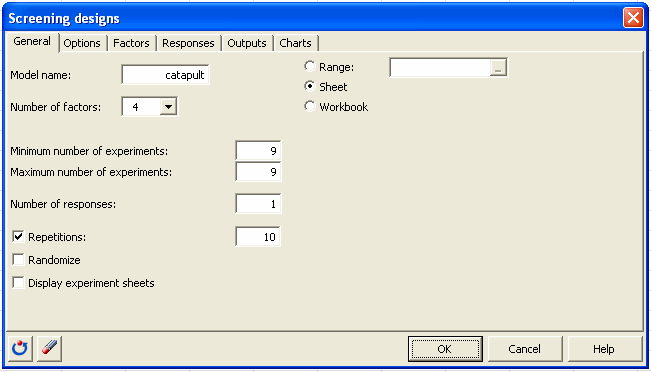In the „Options“ tab, you can keep the default values. In the “factors” tab, select the corresponding columns in the Excel sheet “Sheet1” as shown in the screenshot in order to enter the information about the factors: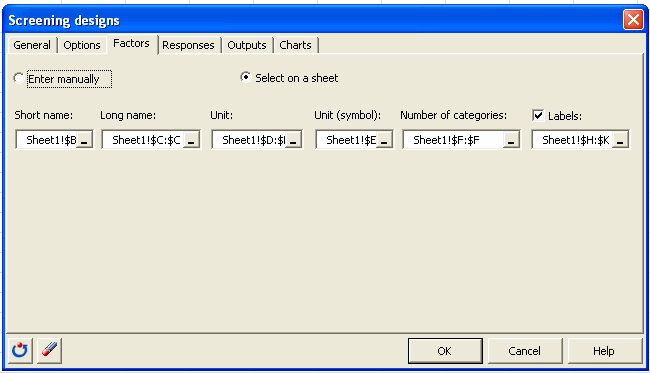In the “responses” tab enter the information about the response variable.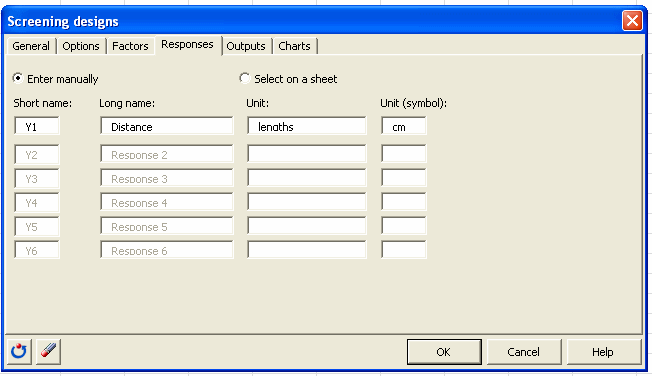Once you have clicked on the “Ok” button, the computation starts. A new window is displayed with orthogonal designs from the internal data base that are closely related to the problem. All designs that have a distance value of 0 fit exactly the described problem with in this case a Latin Square Design. In our example we chose the proposed solution.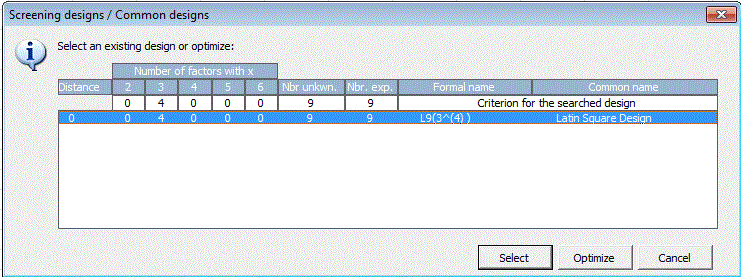A table with information about the factors of the experimental design and the design table itself are displayed in the Excel sheet catapult.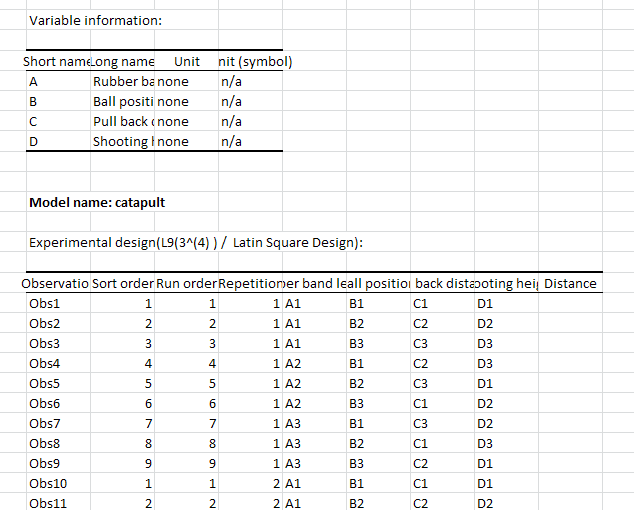## 2. Step: Carrying out the experiments.

Now the 90 experiments are carried out and the resulting distance is written in the corresponding Excel cell in the generated experimental design.

In the attached file, the results are already entered in order to be able to continue with the analysis. The results are on a yellow background in the file to find them easier.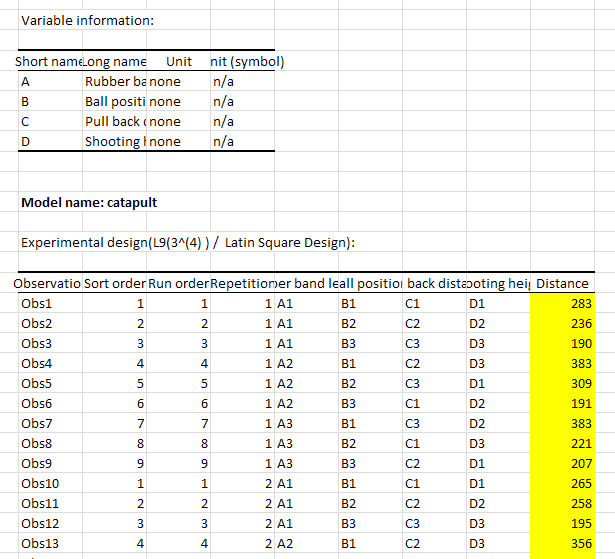## 3. Step: Analysis of the experiments using an ANOVA

After opening XLSTAT, click the DOE button in the ribbon and select “Analysis of a screening design” (see below).Once you've clicked on the button, the dialog box appears. Select the data on the Excel sheet.

Now you are able to select the data in the Excel sheet. Select the name of the model by selection the corresponding cell B25 in the Excel sheet of the experimental design (hier catapult!\$B\$25). By the help of this selection XLSTAT can find information about the chosen experimental planning in the hidden Excel sheet and will use this information during the analysis. Select the result column as shown in the screenshot below.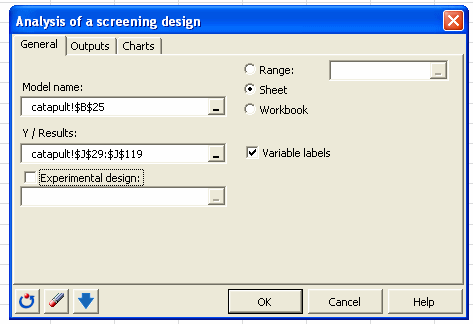In the „Output“ tab, activate only the most important information for this tutorial. You can redo the analysis and select more options in order to have additional information in the report.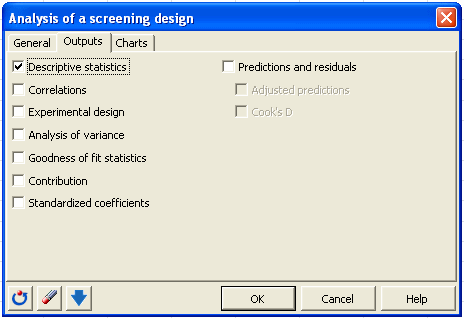Once you have clicked on the “Ok” button, the computation starts.

The first result is a table with the descriptive statistics about the 90 experiments. Then the goodness of fit coefficients R2 and Q2 are displayed. A value close to 1 indicates that the model has a good fitting to the data. This ANOVA and its model describe the data very well, because R2 =  0,894.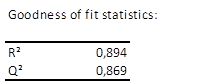Further details about the model are available in the two following sections with the model parameters and the model equation. The most important for the study of the factor effects are the mean chart: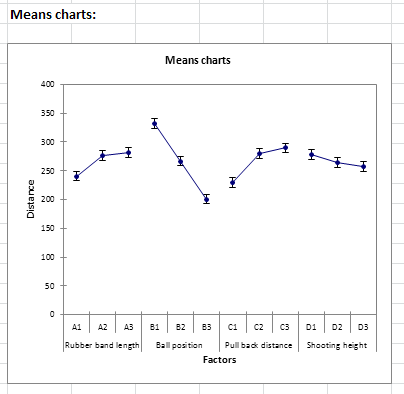In this diagram the effect of each factor with respect to the distance is displayed. For a given factor the mean distance for each of its categories is displayed and connected by a line. The factor with the biggest variation has the biggest effect. In this example it is the factor “ball position” with a distance varying between 331 for B1 and 200 for B3. The smallest effect has the factor “shooting height” with 277 for D1 and 257 for D3. By the help of the repeated measurements for each of the categories, confidence intervals can be calculated and displayed around each mean value.

As a conclusion of the tutorial, the factor “shooting height” has only little influence compared to the other factors and can be taken out of the analysis.

The factor “ball position” should be analyzed in a closer way in order to find the optimal position. This could be done for instance together with the two other factors “rubber band length” and “pull back distance” in a surface response experimental design.

1c26995d494fb3061dd0ae8571ffc0a4@xlstat.desk-mail.com
https://cdn.desk.com/
false
desk